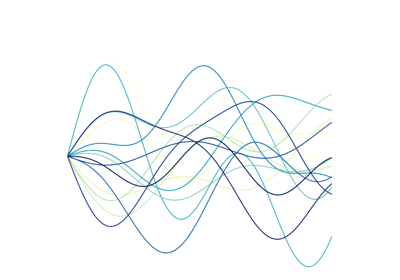# `tslearn.preprocessing`.TimeSeriesScalerMinMax¶

class tslearn.preprocessing.TimeSeriesScalerMinMax(value_range=(0.0, 1.0))[source]

Scaler for time series. Scales time series so that their span in each dimension is between `min` and `max` where `value_range=(min, max)`.

Parameters
value_rangetuple (default: (0., 1.))

The minimum and maximum value for the output time series.

Notes

This method requires a dataset of equal-sized time series.

NaNs within a time series are ignored when calculating min and max.

Examples

```>>> TimeSeriesScalerMinMax(value_range=(1., 2.)).fit_transform([[0, 3, 6]])
array([[[1. ],
[1.5],
[2. ]]])
>>> TimeSeriesScalerMinMax(value_range=(1., 2.)).fit_transform(
...     [[numpy.nan, 3, 6]]
... )
array([[[nan],
[ 1.],
[ 2.]]])
```

Methods

 `fit`(X[, y]) A dummy method such that it complies to the sklearn requirements. `fit_transform`(X[, y]) Fit to data, then transform it. `get_params`([deep]) Get parameters for this estimator. `set_output`(*[, transform]) Set output container. `set_params`(**params) Set the parameters of this estimator. `transform`(X[, y]) Will normalize (min-max) each of the timeseries.
fit(X, y=None, **kwargs)[source]

A dummy method such that it complies to the sklearn requirements. Since this method is completely stateless, it just returns itself.

Parameters
X

Ignored

Returns
self
fit_transform(X, y=None, **kwargs)[source]

Fit to data, then transform it.

Parameters
Xarray-like of shape (n_ts, sz, d)

Time series dataset to be rescaled.

Returns
numpy.ndarray

Resampled time series dataset.

get_params(deep=True)[source]

Get parameters for this estimator.

Parameters
deepbool, default=True

If True, will return the parameters for this estimator and contained subobjects that are estimators.

Returns
paramsdict

Parameter names mapped to their values.

set_output(*, transform=None)[source]

Set output container.

See Introducing the set_output API for an example on how to use the API.

Parameters
transform{“default”, “pandas”}, default=None

Configure output of transform and fit_transform.

• “default”: Default output format of a transformer

• “pandas”: DataFrame output

• None: Transform configuration is unchanged

Returns
selfestimator instance

Estimator instance.

set_params(**params)[source]

Set the parameters of this estimator.

The method works on simple estimators as well as on nested objects (such as `Pipeline`). The latter have parameters of the form `<component>__<parameter>` so that it’s possible to update each component of a nested object.

Parameters
**paramsdict

Estimator parameters.

Returns
selfestimator instance

Estimator instance.

transform(X, y=None, **kwargs)[source]

Will normalize (min-max) each of the timeseries. IMPORTANT: this transformation is completely stateless, and is applied to each of the timeseries individually.

Parameters
Xarray-like of shape (n_ts, sz, d)

Time series dataset to be rescaled.

Returns
numpy.ndarray

Rescaled time series dataset.

## Examples using `tslearn.preprocessing.TimeSeriesScalerMinMax`¶Nearest neighbors

Nearest neighborsHyper-parameter tuning of a Pipeline with KNeighborsTimeSeriesClassifier

Hyper-parameter tuning of a Pipeline with KNeighborsTimeSeriesClassifierSoft-DTW weighted barycenters

Soft-DTW weighted barycentersSVM and GAK

SVM and GAKLearning Shapelets

Learning ShapeletsAligning discovered shapelets with timeseries

Aligning discovered shapelets with timeseriesLearning Shapelets: decision boundaries in 2D distance space

Learning Shapelets: decision boundaries in 2D distance space